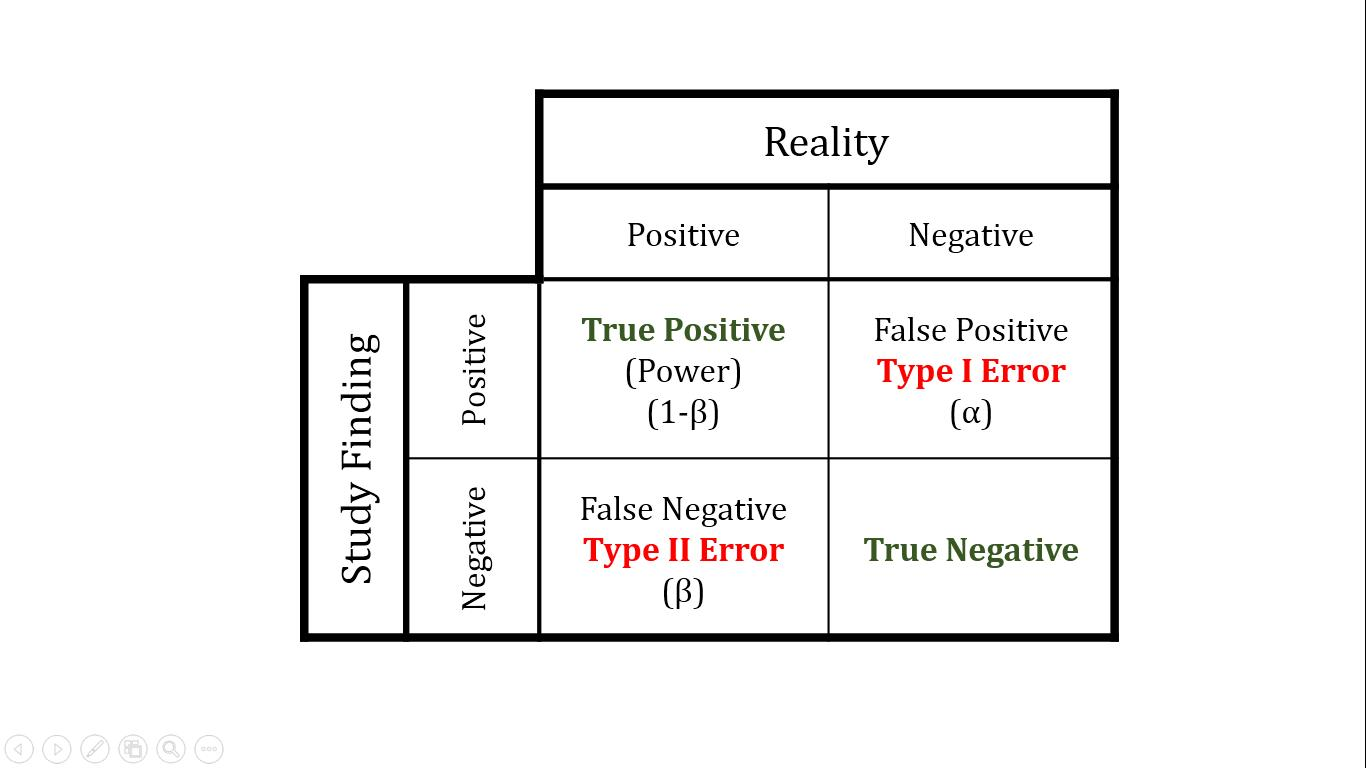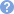Updated: 9/15/2019

# Statistical Hypotheses and Error

0%
Topic
Review Topic
0
0
N/A
N/A
Questions
1
0
0
Topic
 HypothesesNull Hypothesis (H0) Hypothesis of no difference i.e. There is no link between disease and risk factor Alternative Hypothesis (H1) Hypothesis of difference i.e. There is a link between disease and risk factor
 Type I error (False positive) Stating there is an association when none exits Incorrectly rejecting null hypothesis α = probability of type I error p = probability that results as or more extreme than those of the study would be observed if the null hypothesis were trueGeneral rule of thumb is that statistical significance is reached if p ≤ 0.05 Type II error (False negative) Stating there is no effect when an effect exists Incorrectly accepting null hypothesis β = probability of type II error Power (True Positive) Probability of correctly rejecting null hypothesis Power = 1 - β Power depends on Sample size Increasing sample size increases power Size of expected effect Increasing effect size increases power True Negative Probability of correctly accepting null hypothesis

Average 4.5 of 2 Ratings

Questions (1)

(M2.OMB.18.1) In a study, 2 groups are placed on different statin medications, statin A and statin B. Baseline LDL levels are drawn for each group and are subsequently measured every 3 months for 1 year. Average baseline LDL levels for each group were identical. The group receiving statin A exhibited an 11 mg/dL greater reduction in LDL in comparison to the statin B group. Statistical analysis reports a p-value of 0.052. Which of the following best describes the meaning of this p-value?

QID: 210445
1

If 100 similar experiments were conducted, 5.2 of them would show similar results

6%

(1/16)

2

There is a 5.2% chance that A is more effective than B is due to chance

38%

(6/16)

3

There is a 94.8% chance that the difference observed reflects a real difference

19%

(3/16)

4

This is a statistically significant result

25%

(4/16)

5

Though A is more effective than B, there is a 5.2% chance the difference is due to a true effect

12%

(2/16)

M 5 C

Select Answer to see Preferred Response